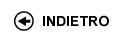Politecnico di Torino
03KWYLI, 03KWYJM, 03KWYLJ, 03KWYLL, 03KWYLM, 03KWYLS, 03KWYLX, 03KWYLZ, 03KWYMA, 03KWYMB, 03KWYMC, 03KWYMH, 03KWYMK, 03KWYMO, 03KWYMQ, 03KWYOD, 03KWYPC, 03KWYPI, 03KWYPL
Geometry
Corso di Laurea in Ingegneria Dell'Autoveicolo (Automotive Engineering) - Torino
Corso di Laurea in Ingegneria Meccanica (Mechanical Engineering) - Torino
Corso di Laurea in Ingegneria Delle Telecomunicazioni (Telecommunications Engineering) - Torino
Espandi...
 Docente Qualifica Settore Lez Es Lab Tut Anni incarico Carlini EnricoO2 MAT/03 60 40 0 0 3 Gatto LetterioAC MAT/03 60 40 0 0 2
 SSD CFU Attivita' formative Ambiti disciplinari MAT/03 10 A - Di base Matematica, informatica e statistica
Esclusioni:
15BCG
 Presentazione The course Geometry has two main objectives. The first aims at teaching students how to analyse a problem, so to clearly distinguish: the initial data (the assumptions), the goals, and the deductive path from the hypotheses to the result (the proof). The basics of Linear Algebra are particularly suited to this end, given the limited number of assumptions usually involved and the simple reasoning that leads to the result. The second objective is to present an array of topics spread over Linear Algebra, Elementary Geometry, Differential Geometry and the Theory of Multivariable Functions, which thus build a bridge to the Analysis I and II courses. Part of the subject matter is in fact dedicated to extending differential calculus from one to several real variables, and touches upon the basic applications. Moreover, the Geometry of Euclidean space is a necessary tool for integral calculus, as treated in Analysi II. Risultati di apprendimento attesi Ability to follow proofs; understanding of the essential properties of elementary geometry of space, the basics of vector spaces plus continuity and differentiability of multivariable functions. Prerequisiti / Conoscenze pregresse Real and complex numbers, trigonometry, equations and inequalities of degree one and two, differential calculus in one variable. Programma VECTORS. The notion of a vector and operations. Dot product, distances, angles. Cross (wedge) product, triple (mixed) product, areas and volumes. Geometry of the plane, lines and conics. Equations of a plane in space. lines in parametric form. MATRICES AND SYSTEMS. Matrices, sum and product of matrices. Powers and inverses of square matrices. Systems of equations, matrix form of a system. Rouche'-Capelli theorem. Linear independence in Rn. Linear combinations and subspaces spanned by vectors. Subspaces in Rn, bases. Spaces generated by rows or columns of matrices. LINEAR MAPS. The notion of vector space. Linear maps of vector spaces. Isomorphisms. Matrices associated to linear maps. Kernel and image. Rank and nullity. Operations of subspaces. Sum and direct sum of subspaces. DIAGONALISATION. Endomorphisms, change of bases. Eigenvalues, determinant of a square matrix. Eigenvectors, subspaces, multiplicity. Symmetric matrices and quadratic forms. Orthogonal matrices. Diagonalisability of square and symmetric matrices. DIFFERENTIAL CALCULUS. Curves in space. Tangent vector and tangent line. Length of a curve and arc length. Functions over Rn. Rudiments of topology. Limits and continuity. Partial derivatives and gradient. Vector-valued functions. Jacobian matrix. FUNCTIONS OF TWO VARIABLES The graph of a map in two variables. Paraboloids. Differentiability and tangent plane. Second derivatives, Hessian matrix. Expansions in Taylor series. realtive maxima and minima. Critical points. SURFACES AND QUADRICS. Surfaces of revolution, spheres. Ellipsoids, hyperboloids. Rotations in the plane and in space. Quadrics, lines, planes. Parametric surfaces. Normal vector, tangent plane and normal line. Organizzazione dell'insegnamento The course consists of lectures and exercises classes in teams. Testi richiesti o raccomandati: letture, dispense, altro materiale didattico The textbooks, chosen among the ones listed, will be recommended during the lectures. 1) S. Greco, P. Valabrega , Lezioni di Geometria , Vol. I Algebra lineare, Vol. 2 Geometria Analitica, Ed. Levrotto e Bella , Torino 2009. 2) C. Cumino, G. Tedeschi, G. Viola, Appunti di Geometria Analitica e Algebra Lineare, Progetto Leonardo, Bologna. 3) A. Sanini, Lezioni di Geometria, Ed. Levrotto e Bella, Torino 1993. 4) A. Sanini, Esercizi di Geometria, Ed. Levrotto e Bella, Torino 1993. Useful material may be found online, either on the website of the Politecnico di Torino or on the pages of the single courses. Criteri, regole e procedure per l'esame The final evaluation will be made with a written examination. However, an oral colloquium can be requested by the teacher. Orario delle lezioni Statistiche superamento esami Programma definitivo per l'A.A.2012/13© Politecnico di Torino
Corso Duca degli Abruzzi, 24 - 10129 Torino, ITALY